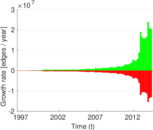# Amazon

These are ratings from Amazon.

 Code `AM` Internal name `amazon` Name Amazon Data source http://jmcauley.ucsd.edu/data/amazon/ Availability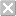Dataset is not available for download Consistency checkDataset passed all tests Category Rating network Dataset timestamp 1996-05 ⋯ 2014-07 Node meaning User, product Network formatBipartite, undirected Edge type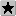Ratings, no multiple edges Temporal dataEdges are annotated with timestamps

## Statistics

 Size n = 31,050,733 Left size n1 = 21,176,522 Right size n2 = 9,874,211 Volume m = 82,677,131 Wedge count s = 25,196,597,662 Claw count z = 67,889,727,718,556 Cross count x = 356,886,775,500,116,544 Maximum degree dmax = 44,557 Maximum left degree d1max = 44,557 Maximum right degree d2max = 25,368 Average degree d = 5.325 29 Average left degree d1 = 3.904 19 Average right degree d2 = 8.373 04 Fill p = 3.953 92 × 10−7 Size of LCC N = 30,767,837 Diameter δ = 24 50-Percentile effective diameter δ0.5 = 5.615 55 90-Percentile effective diameter δ0.9 = 6.962 84 Median distance δM = 6 Mean distance δm = 6.132 91 Gini coefficient G = 0.704 302 Balanced inequality ratio P = 0.223 299 Left balanced inequality ratio P1 = 0.260 054 Right balanced inequality ratio P2 = 0.182 262 Relative edge distribution entropy Her = 0.913 027 Power law exponent γ = 2.309 95 Tail power law exponent γt = 2.351 00 Degree assortativity ρ = −0.016 024 2 Degree assortativity p-value pρ = 0.000 00 Spectral norm α = 176.257 Spectral separation |λ1[A] / λ2[A]| = 1.124 04 Negativity ζ = 0.350 228

## Plots

### Degree distribution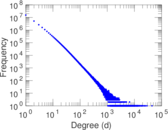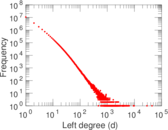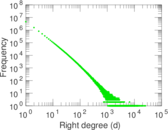### Cumulative degree distribution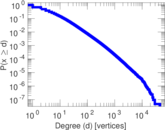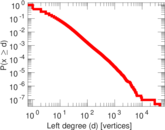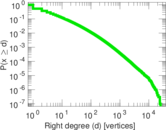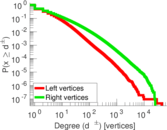### Lorenz curve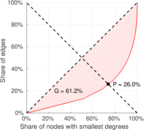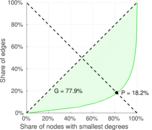### Spectral distribution of the adjacency matrix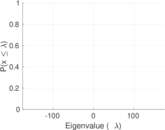### Spectral distribution of the normalized adjacency matrix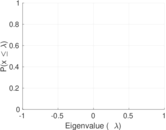### Spectral distribution of the Laplacian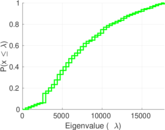### Spectral graph drawing based on the adjacency matrix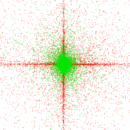### Spectral graph drawing based on the normalized adjacency matrix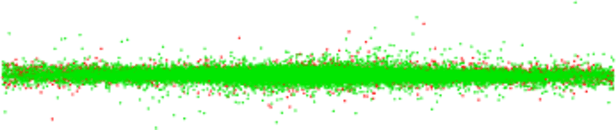### Degree assortativity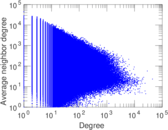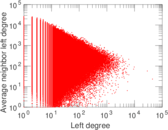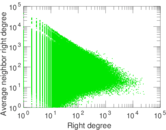### Zipf plot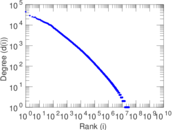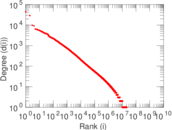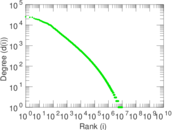### Hop distribution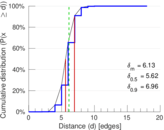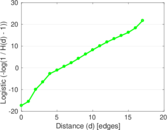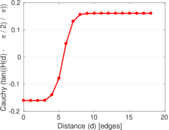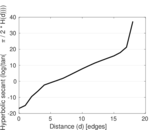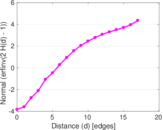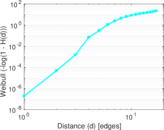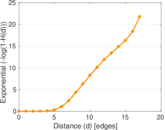### Edge weight/multiplicity distribution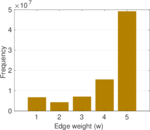### Temporal distribution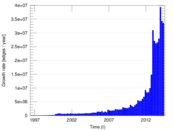### Signed temporal distribution